# Optical models¶

## Photon energy¶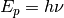with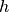Planck constant and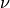is photon frequency.

## Coherent transfer matrix method¶

Transfer matrix method a convenient way of modeling thin film stacks. It is assumed that layers are stacked along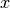axis, with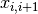being interface between layerand layer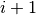. Optical properties of each layer are specified by wavelength dependent complex refraction coefficient.

Optical field inside layerat given point alongaxis is specified by column vector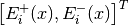, with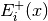being complex amplitude of forward traveling wave, and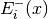being complex amplitude of backward traveling wave.

Snell law is determines angles of propagation in each layer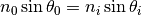where index 0 refers to medium before first layer.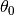is angle of illuminating wave. All angles can be complex numbers. Since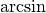is multivalued function, angle of forward traveling wave is found from conditions that forward wave has forward pointing Poynting vector, or alternatively, that the amplitude of forward wave decays in absorbing medium.

In this convention, interface between layers is described by matrix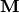as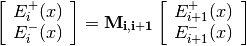with entries of matrixspecified as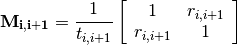where transmission coefficient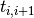and reflection coefficient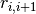are given by Fresnel equations for complex amplitudes of light passing from layer i to layer i+1. Coefficients for backward propagating wave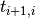and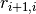are eliminated using Stokes relations.

For s-polarized wave: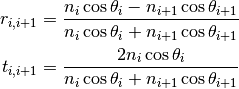For p-polarized wave: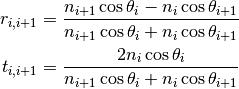Propagation inside layer is described by matrix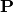as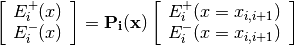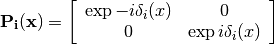with phase-shift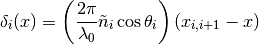Light entering layer, on side of layer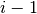has vector of complex amplitudes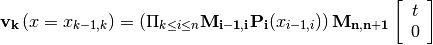with vector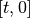denoting light leaving the device on the side opposite to illumination, withbeing complex amplitude of transmitted wave.

Applying above to whole device gives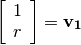with amplitude of illuminating wave set arbitrarily toand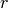being complex amplitude of reflected wave.

When analyzing stack, firstly, solution,is found. Then intensity of light anywhere inside the device is calculated using found vectors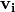and propagation matrices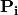. Total intensity is found by applying Poynting formula. Absorbed energy is found by differentiating with respect to.

## Incoherent light¶

Incoherent light is described by spectrum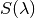. Absorption of incoherent light is calculated as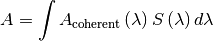where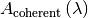is calculated using coherent transfer matrix method.